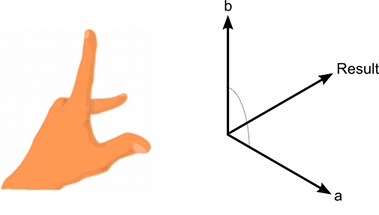Legacy Documentation: Version 5.4
LanguageEnglish
• C#
• JS

## Script language

Select your preferred scripting language. All code snippets will be displayed in this language.

# Vector3.Cross

Suggest a change

## Success!

Thank you for helping us improve the quality of Unity Documentation. Although we cannot accept all submissions, we do read each suggested change from our users and will make updates where applicable.

Close

## Sumbission failed

For some reason your suggested change could not be submitted. Please try again in a few minutes. And thank you for taking the time to help us improve the quality of Unity Documentation.

Close
public static function Cross(lhs: Vector3, rhs: Vector3): Vector3;
public static Vector3 Cross(Vector3 lhs, Vector3 rhs);

## Description

Cross Product of two vectors.

The cross product of two vectors results in a third vector which is perpendicular to the two input vectors. The result's magnitude is equal to the magnitudes of the two inputs multiplied together and then multiplied by the sine of the angle between the inputs. You can determine the direction of the result vector using the "left hand rule".The left hand rule applied to Cross(a, b).

```	// Get the normal to a triangle from the three corner points, a, b and c.
function GetNormal(a: Vector3, b: Vector3, c: Vector3) {
// Find vectors corresponding to two of the sides of the triangle.
var side1 = b - a;
var side2 = c - a;

// Cross the vectors to get a perpendicular vector, then normalize it.
return Vector3.Cross(side1, side2).normalized;
}```
```using UnityEngine;
using System.Collections;public class ExampleClass : MonoBehaviour {
Vector3 GetNormal(Vector3 a, Vector3 b, Vector3 c) {
Vector3 side1 = b - a;
Vector3 side2 = c - a;
return Vector3.Cross(side1, side2).normalized;
}
}
```